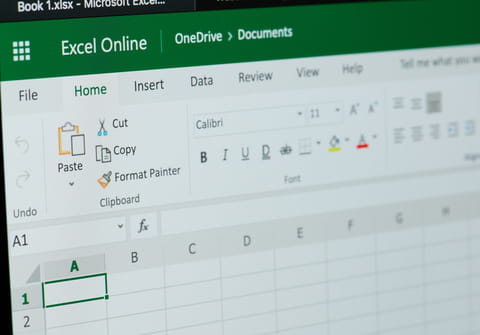# Convert numbers to words in Excel: without VBA, formulaThis article will teach you how to convert a numeric value in a Microsoft Excel worksheet cell into words using what is called the Spell Number function.

## How to convert numbers to words in Excel?

• Without VBA

The first method for converting a number to its written equivalent is to use direct entry. For example, if you were looking to write out 47.75 into forty seven dollars and seventy five cents, you can do this by entering the following formula into a cell:

=SpellNumber(47.75)

• Insert function

Another way to change numbers to text is to use Insert Function. To do this, select the cell that you want, and then, click Insert Function on the Formulas ribbon.

Under Or, select a category, followed by User Defined. In the Select a function list, select SpellNumber, followed by OK.

Next, enter the number or cell reference that you want, and then click OK.

• Concatenate function

Alternatively, you can use the Concatenate function to apply this formula to specific cells (though this method is a bit more complicated). To use this function, enter the code below (be sure to replace B6 with the applicable cell number):

```=CONCATENATE(TEXT(UPPER(Spell Number(B6)),0),"ONLY")
```
Need more help with Excel? Check out our forum!
Subject Replies